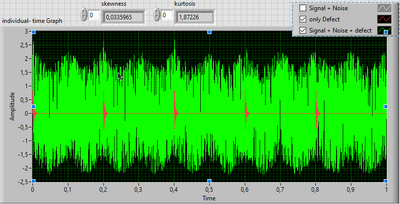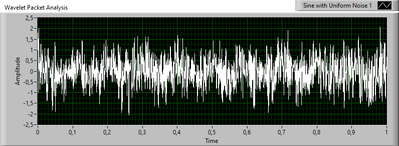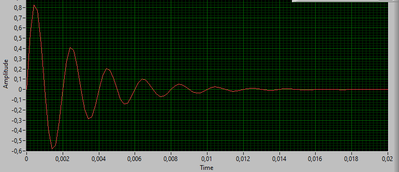# LabVIEW

cancel
Showing results for
Did you mean:

## Wavelet Transformation- Advanced signal processing

Goal: To find the defect frequencies of the bearing (rolling-element) from the "simulated" acceleration signal.

I am trying to use "Wavelet transform" functions of Advanced signal processing toolkit for the defect identification.

Below shown is the acceleration signal in green (cosine of 10 Hz frequency), which has noise and defect signal (in RED plot, having 5 Hz frequency). I tried to get back the red plot with the help of Wavelet analysis, but was not possible as it is hard for me to comprehend these wavelet functions.Below is the graph showing what I have got in (a) time domain and in (b) frequency domainthe above graph should get closer to the defect (Red plot shown in the first graph) signal.I have used 1. BP Filter (see below for config.) -> white Plot2. Wavelet packet analysis tool (see  below for config.) -> red Plot. used db143. Band pass filter (IIR filter with LPF- 3 Hz and HPF-7 Hz (since i know my defect frequency!))-> Green plot (PERFECT!!  I need this but from WPT analysis with that fact that defect frequency is unkown, is that possible ?)

4. Wavelet denoise  (see  below for config.) -> Blue Plot. Just used Bior3_X, since it was more closer to the defect waveform shape than daubechies.I know that to find the defect signal, I need to use a mother wavelet which is very similar to the defect waveform. But I dont know to choose that. below is my defect signal with 500Hz frequency, which is repeating at 5 Hz.I have attached the labview file (saved as html, if any trouble opening it, let me know) that I developed along with the  acceleration signal dataset (with 3 Y-axes columns- Signal + noise, defect, 1st and 2nd columns superimposed) .

I try to organise the question as clear as possible, but I can do more if you cannot understand my points.

Message 1 of 2
(224 Views)

## Re: Wavelet Transformation- Advanced signal processing

Hello Everyone,

as I did not get any reply for my post, I ask doubts regard to more concrete implementation of Wavelet tools.

Wavelet packet analysis: How do I get wavelet denoised signal for each of the node levels. For eg., I used Wavelet: db04 with Level: 3 then there are 8 nodes possible 3.0, 3.1, 3.2, ... 3.7, then I do I get the signala for each of these 8 nodes. When I display the node coefficients (refer to my block diagram in my precious post), i get a (only one) co-effciient of -0,661829 for node "0". What does this number signify?

Also, is it possible to define a "morely" mother wavelet in labview when I know the center frequency(fc) and bandwidth (sigma)? IF so, let me know. And which tool to use to filter my signal with this wavelet ? any eg. implementation.